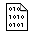## Firefly and PC GAMESS-related discussion club

Learn how to ask questions correctlyGeometry optimization problem with MCSCF with ECP in C3 symmetry.

Alex Nest

Good morning!

We have very strange problem with MCSCF geometry optimization of Fe3S4 cluster (it is kind of protein Fe-S clusters). We have done the following things:

1) first we know (from refs) that our cluster with charge -2 has multiplicity 2.
2) we selected the basis 6-31G** (non-Fe) / LANL2DZ (Fe) and prepare input for C3 symmetry
3) we tried to perform ROHF, but we could not converge it. Only UHF converges but very hard and with very high value of S-SQUARED (~ 6.0 that indicates very large spin contamination). We decided that ground state could be multi-reference and decided to perform MCSCF.
4) we remove one electron and converge RHF for such system
5) then we look at orbitals and selected (3,3) active space
6) then we take vector from (4) step and start MCSCF convergency (it was not hard).
7) then we started MCSCF geometry optimization and faces the following problem:
rms grew:
but energy decreased:
NSERCH=  0     ENERGY=   -3269.0048828
NSERCH=  1     ENERGY=   -3269.0154026
NSERCH=  2     ENERGY=   -3269.0019545
NSERCH=  3     ENERGY=   -3268.9884073
NSERCH=  4     ENERGY=   -3268.9871148
NSERCH=  5     ENERGY=   -3268.9903808
NSERCH=  6     ENERGY=   -3268.9930019
NSERCH=  7     ENERGY=   -3268.9968767
NSERCH=  8     ENERGY=   -3268.9989956
NSERCH=  9     ENERGY=   -3269.0010380
NSERCH= 10     ENERGY=   -3269.0028638
NSERCH= 11     ENERGY=   -3269.0016070
NSERCH= 12     ENERGY=   -3269.0037472
NSERCH= 13     ENERGY=   -3269.0063914
NSERCH= 14     ENERGY=   -3269.0094045
NSERCH= 15     ENERGY=   -3269.0083837
NSERCH= 16     ENERGY=   -3269.0083270
NSERCH= 17     ENERGY=   -3269.0083347
NSERCH= 18     ENERGY=   -3269.0083693
NSERCH= 19     ENERGY=   -3269.0064975
8) we tried SA-MCSCF (adding wstate=1,0.1 to \$DET and ntrack=2 to \$MCSCF) but approximately the same picture was obtained.

Firefly v.8.1.1 was used. Can you advice something in our case? Could you tell if our modelling strategy is right in global?

Attached please see the input of step (7).This message contains the 1403 kb attachment [ casopt.inp ] MCSCF optimization from step (7)Thu Aug 11 '16 1:06amThis message read 600 times